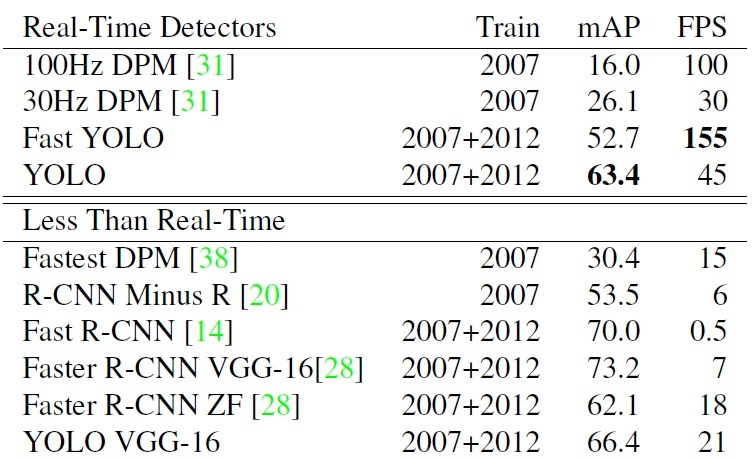## 2 YOLOv1

### 2.1 滑动窗口与CNN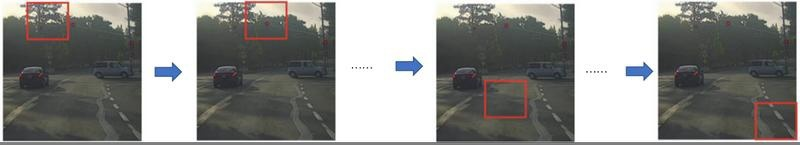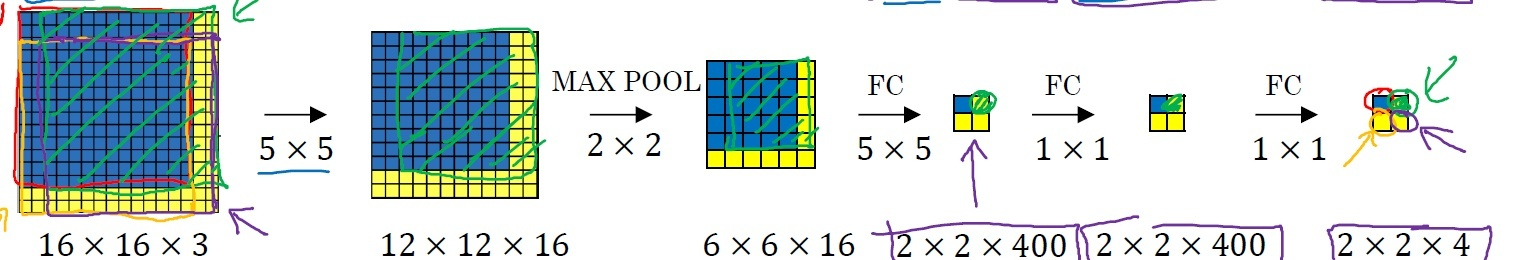### 2.2 设计理念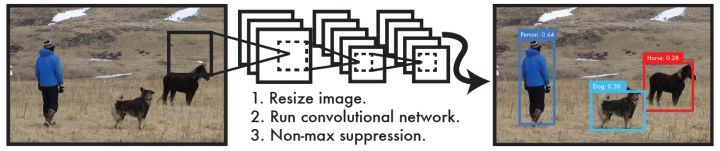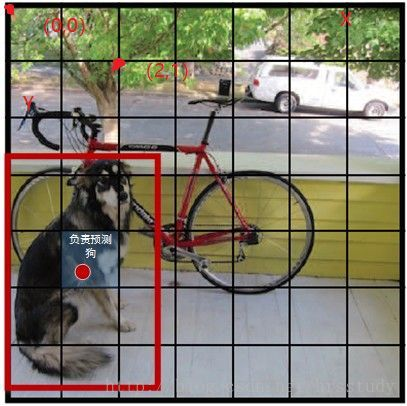1. 这个边界框含有目标的可能性大小：$$Pr(object)$$ 当该边界框是背景时（即不包含目标），此时 $$Pr(object)=0$$ 。而当该边界框包含目标时， $$Pr(object)=1$$ 。
2. 这个边界框的准确度：边界框的准确度可以用预测框与实际框（ground truth）的IOU（intersection over union，交并比）来表征，记为 $$\text{IOU}^{truth}_{pred}$$ 。

$$Pr(class_{i}|object)*Pr(object)*\text{IOU}^{truth}_{pred}=Pr(class_{i})*\text{IOU}^{truth}_{pred}$$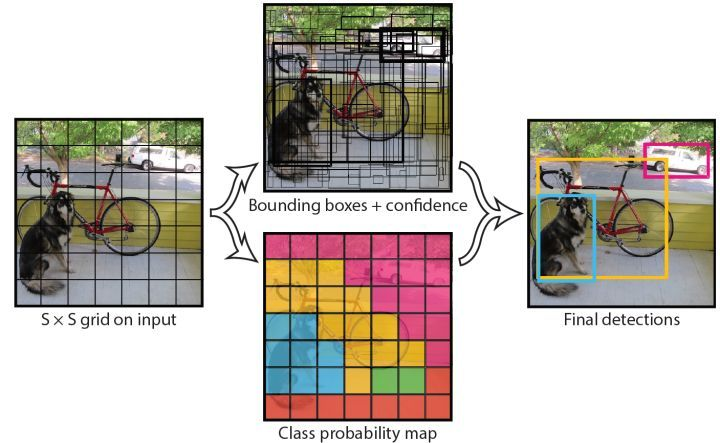### 2.3 网络设计

Yolo采用卷积网络来提取特征，然后使用全连接层来得到预测值。网络结构参考GooLeNet模型，包含24个卷积层和2个全连接层，如图8所示。对于卷积层，主要使用1x1卷积来做channle reduction，然后紧跟 $$3\times 3$$ 卷积。对于卷积层和全连接层，采用 Leaky ReLU 激活函数： $$max(x, 0.1x)$$ 。但是最后一层却采用线性激活函数。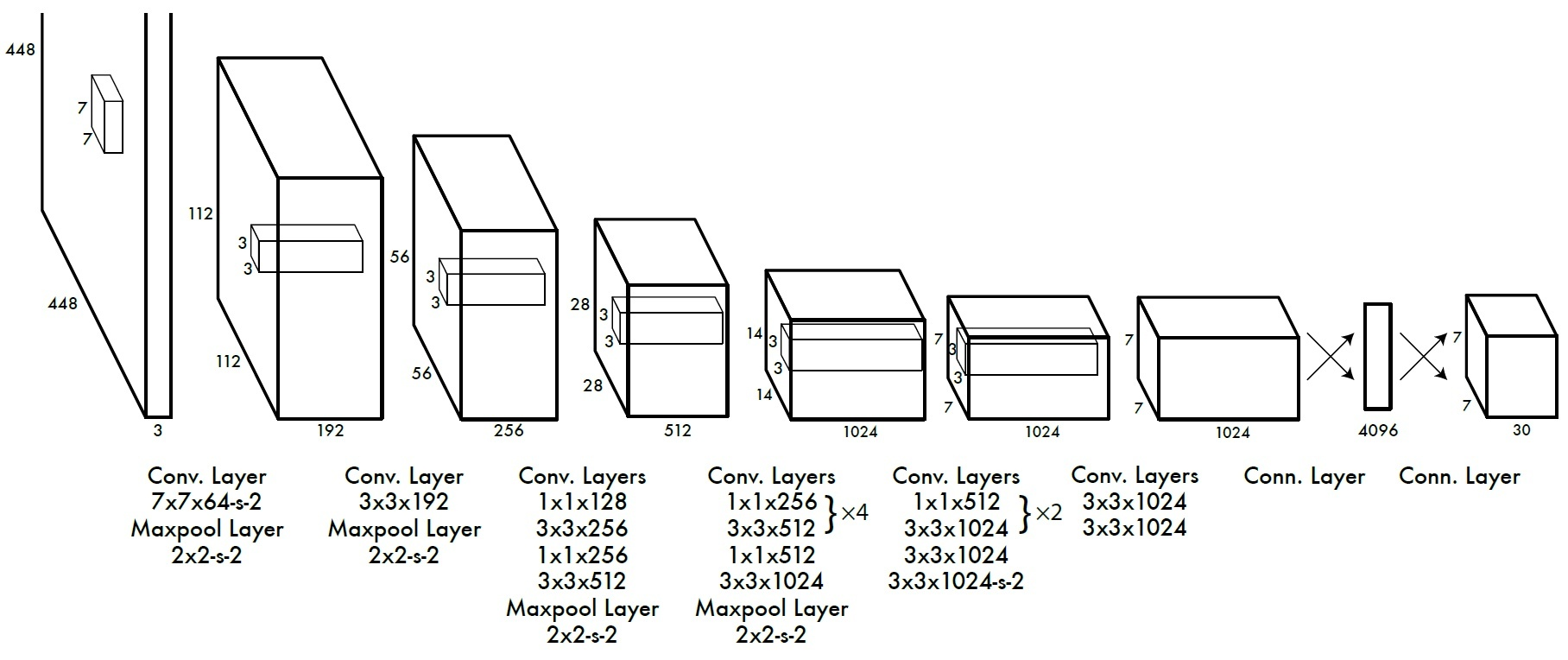• 采用切片，那么 $$P_{[:,\ 0:7*7*20]}$$ 就是类别概率部分
• 而 $$P_{[:,\ 7*7*20:7*7*(20+2)]}$$ 是置信度部分
• 最后剩余部分 $$P_{[:,\ 7*7*(20+2):]}$$ 是边界框的预测结果。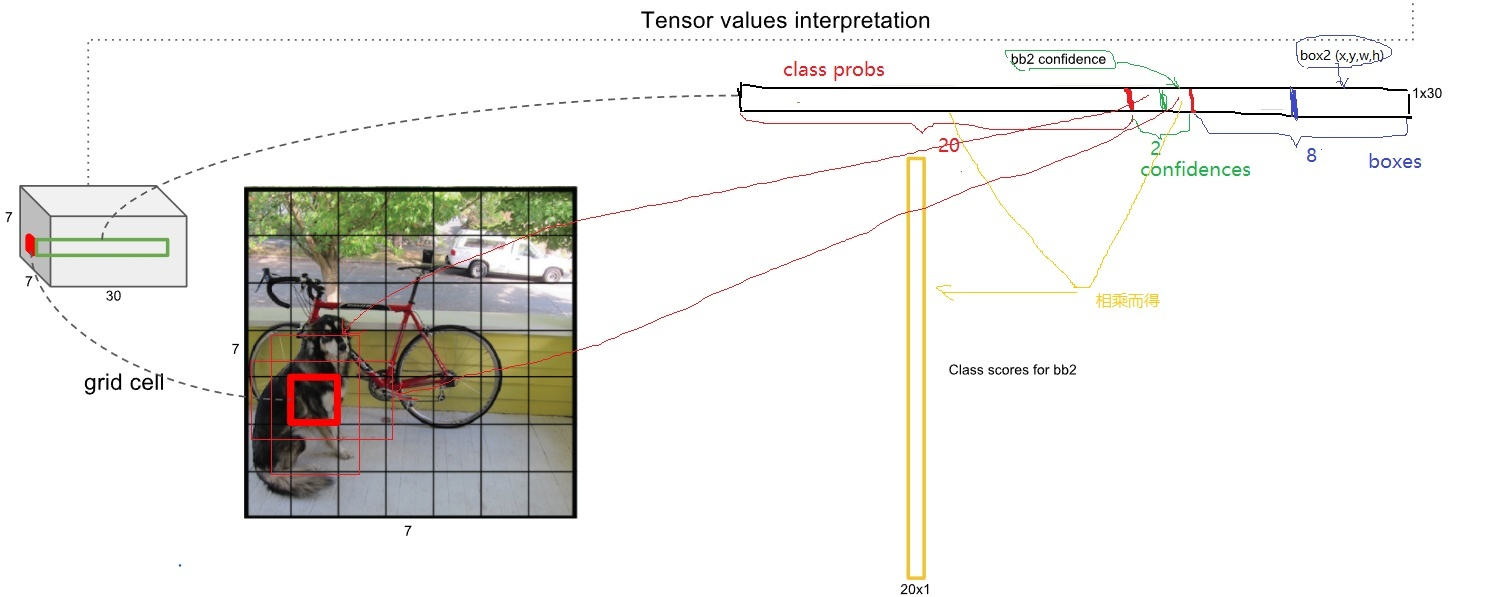def _build_detector(self):
"""Interpret the net output and get the predicted boxes"""
# the width and height of orignal image
self.width = tf.placeholder(tf.float32, name="img_w")
self.height = tf.placeholder(tf.float32, name="img_h")
# get class prob, confidence, boxes from net output
idx1 = self.S * self.S * self.C
idx2 = idx1 + self.S * self.S * self.B
# class prediction
class_probs = tf.reshape(self.predicts[0, :idx1], [
self.S, self.S, self.C])
# confidence
confs = tf.reshape(self.predicts[0, idx1:idx2], [
self.S, self.S, self.B])
# boxes -> (x, y, w, h)
boxes = tf.reshape(self.predicts[0, idx2:], [
self.S, self.S, self.B, 4])

# convert the x, y to the coordinates relative to the top left point of the image
# the predictions of w, h are the square root
# multiply the width and height of image
boxes = tf.stack([(boxes[:, :, :, 0] + tf.constant(self.x_offset, dtype=tf.float32)) / self.S * self.width,
(boxes[:, :, :, 1] + tf.constant(self.y_offset,
dtype=tf.float32)) / self.S * self.height,
tf.square(boxes[:, :, :, 2]) * self.width,
tf.square(boxes[:, :, :, 3]) * self.height], axis=3)

# class-specific confidence scores [S, S, B, C]
scores = tf.expand_dims(confs, -1) * tf.expand_dims(class_probs, 2)

scores = tf.reshape(scores, [-1, self.C])  # [S*S*B, C]
boxes = tf.reshape(boxes, [-1, 4])  # [S*S*B, 4]

# find each box class, only select the max score
box_classes = tf.argmax(scores, axis=1)
box_class_scores = tf.reduce_max(scores, axis=1)

# filter the boxes by the score threshold
filter_mask = box_class_scores >= self.threshold
scores = tf.boolean_mask(box_class_scores, filter_mask)
boxes = tf.boolean_mask(boxes, filter_mask)
box_classes = tf.boolean_mask(box_classes, filter_mask)

# non max suppression (do not distinguish different classes)
# ref: https://tensorflow.google.cn/api_docs/python/tf/image/non_max_suppression
# box (x, y, w, h) -> box (x1, y1, x2, y2)
_boxes = tf.stack([boxes[:, 0] - 0.5 * boxes[:, 2], boxes[:, 1] - 0.5 * boxes[:, 3],
boxes[:, 0] + 0.5 * boxes[:, 2], boxes[:, 1] + 0.5 * boxes[:, 3]], axis=1)
nms_indices = tf.image.non_max_suppression(_boxes, scores,
self.max_output_size, self.iou_threshold)
self.scores = tf.gather(scores, nms_indices)
self.boxes = tf.gather(boxes, nms_indices)
self.box_classes = tf.gather(box_classes, nms_indices)

### 2.4 网络训练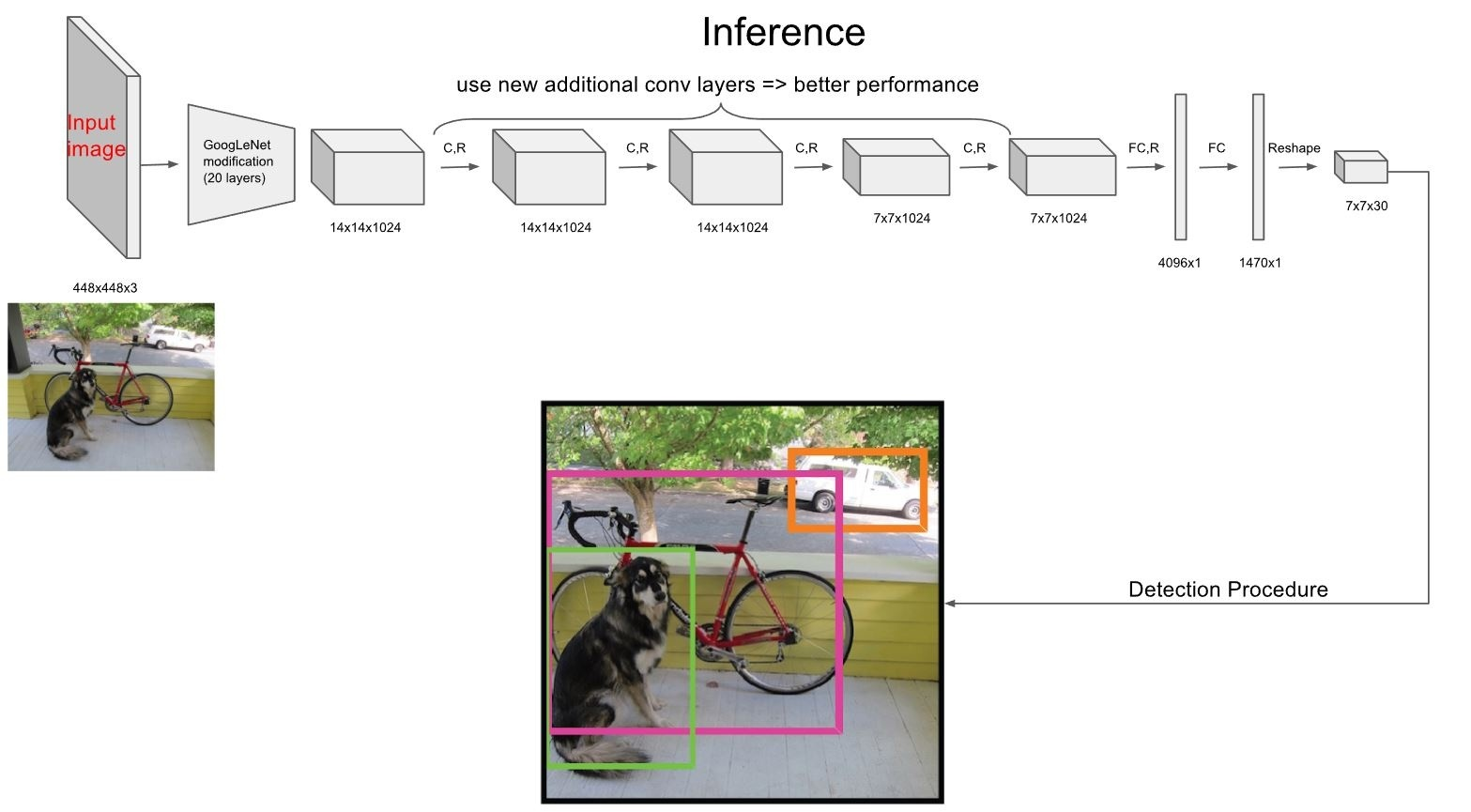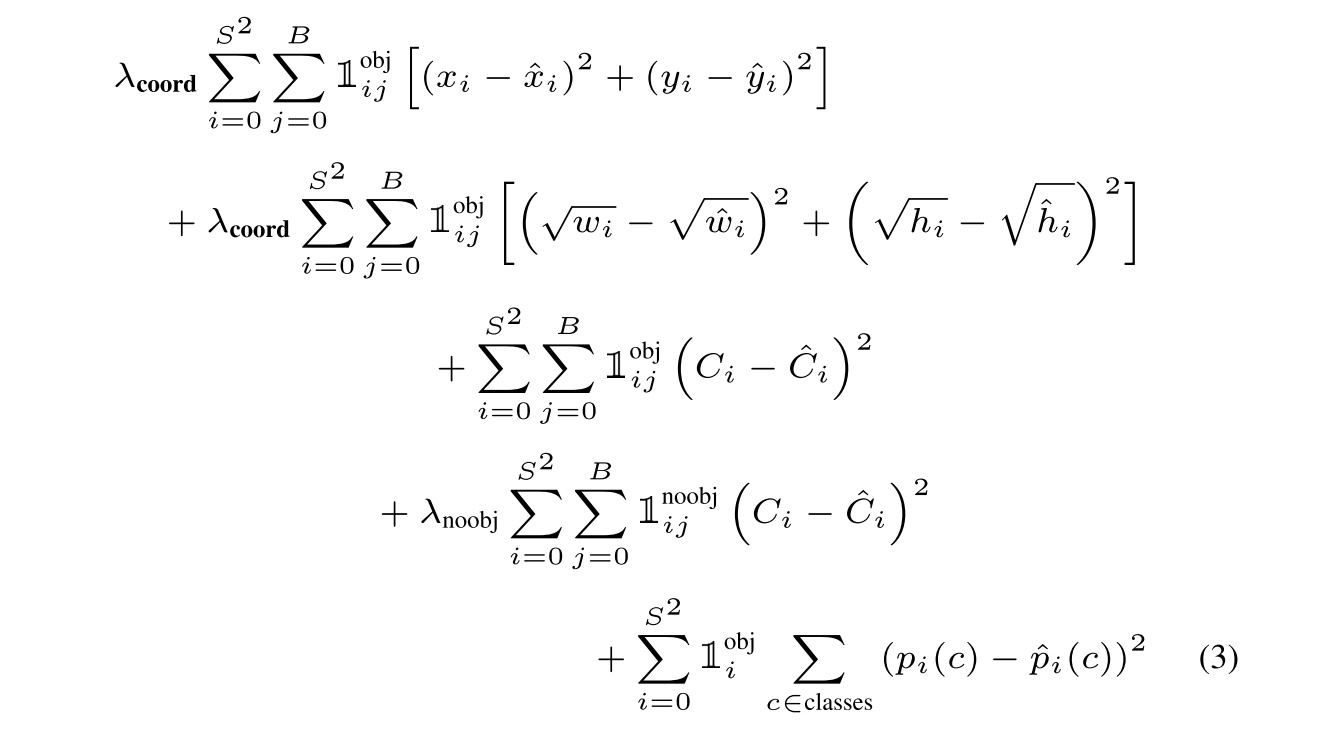• 其中第一项是边界框中心坐标的误差项， $$1^{obj}_{ij}$$ 指的是第 $$i$$ 个单元格存在目标，且该单元格中的第 $$j$$ 个边界框负责预测该目标。
• 第二项是边界框的高与宽的误差项。
• 第三项是包含目标的边界框的置信度误差项。
• 第四项是不包含目标的边界框的置信度误差项。
• 而最后一项是包含目标的单元格的分类误差项， $$1^{obj}_{i}$$ 指的是第 $$i$$ 个单元格存在目标。

### 2.5 网络预测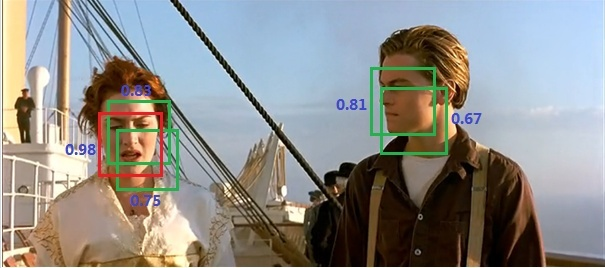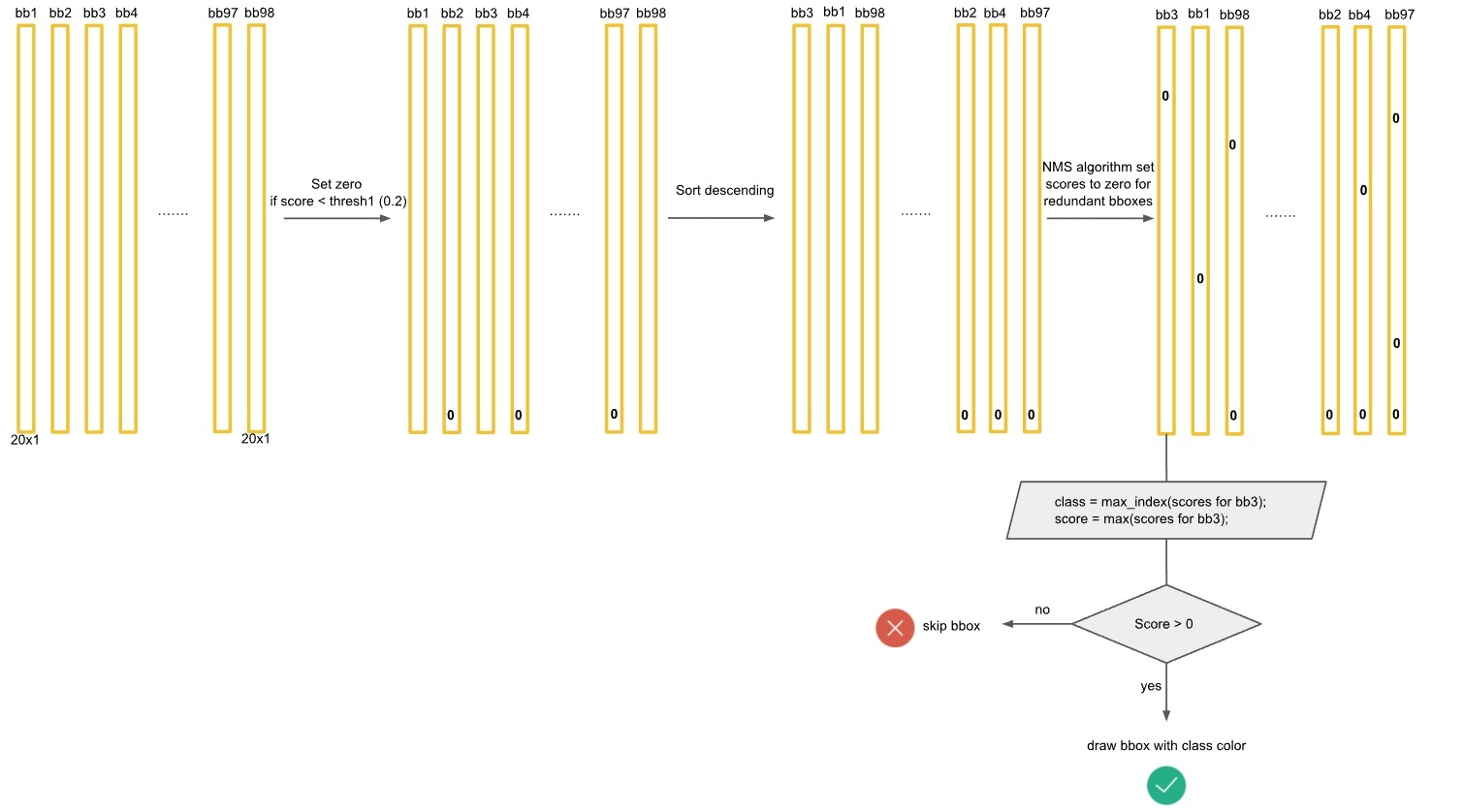Yolo的预测处理流程

### 2.6 算法性能分析